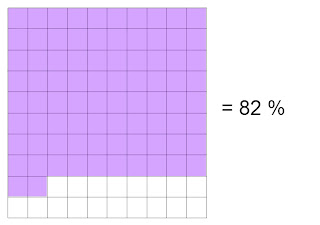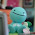## Sunday, January 16, 2011

### Final Percent Post

What is a percent?

-Means out of 100

-Another name for hundreths

-Ex. 76% means 76 out of 100

4.1 Representing Percents

-You can represent a percent by shading in a hundred grid

-You can also use more than one grid to represent a percent larger than 100

-If representing a fractional percent, you can shade in a part of one square

Ex.4.2 Fractions, Decimals, and Percents

-Fractions, decimals, and percents can be used to represent numbers in various situations

-Percents can also be written as fractions and decimals

Ex. 50% = 0.5 = ½

4.3 Percent of a Number

-To find percents of some numbers, you can use mental math strategies such as halving, doubling and dividing by ten

-Write a percent as a decimal and multiply it by the number to find the percent of a number

Ex. 12 ½ % of 50 = 0.125 x 50 = 6.25

4.4 Combining Percents

-You can add some percents in order to solve a problem

-To find the increase of a number,

-You can add the combined percent amount to the original amount

Ex. 19% of 100 = 0.19 x 100 = 19

100 + 19 = 119

- You can multiply the original number by a single percent greater than 100

Ex. 119% of 100 = 1.19 x 100 = 119

1.